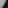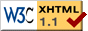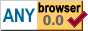DCT - Discrete Cosine Transform

# DCT - Discrete Cosine Transform

2023-03-23

## Introduction

The Discrete Cosine Transform (DCT for short) is a part of many lossy compression formats. It is a reversible transformation between sample values (e.g. pixel data) and DCT coefficients. Bits can be discarded from the DCT coefficients while still allowing the reconstruction of data that is perceptually similar to the original input. The discrete cosine transform is used in many image, video, and audio compression formats, including JPEG, MPEG, and MP3. This article describes how the discrete cosine transform works, using image data as an example.

## Overview

The Discrete Cosine Transform (DCT for short) transforms a signal from the time domain to the frequency domain. In other words, its input is a series of values taken at regular time intervals, and its output is a series of coefficients that represent how well the input correlates with cosine waves at different frequencies. When working with images, instead of time, we use pixel coordinates, but the calculations are the same.

The DCT itself is fully reversible (meaning the original image can be recovered exactly), but the transform lends itself especially well to lossy compression. Getting the DCT coefficients slightly wrong results in an image that still looks like the original, and the DCT tends to produce many coefficients that are close to zero. Storing coefficients in fewer bits than an exact representation would require and/or omitting the zero coefficients can result in great space savings. The major downside to the DCT is that lossy compression tends to result in ringing artifacts, where sharp edges in the image are surrounded by pixels that are far from their correct values.

## Technical Details

The way the DCT transforms a signal from the time domain to the frequency domain is by multiplying the value at each point in time by a value of the cosine function at a related point in time, and summing the results. This produces a single value. We then repeat the process a number of times, each with a larger step size for the cosine function (cycling through the values of the cosine function faster). For n input values, we produce n output values.The cosine values we use are given by cos(π * i / n * (t + ½)), where i denotes the number of the output value we are producing, t denotes time (or pixel coordinate, in case of images), and n denotes the number of samples we will be using. The image on the right shows what the cosine curves look like for n=4. We only use the circled values at t=0,1,2,3. Some observations about the curves and values:

• The curve for i=0 is a horizontal line at value 1.
• For i=1, the values are positive on the left and negative on the right.
• For i=2, the values are positive at the edges and negative in the middle.
• For i=3, the value is strongly negative at t=1 and strongly positive at t=2.

Together, these curves capture changes in the input signal. For example, a large increase between t=1 and t=2 will increase the result of multiplying by the curve for i=3 and decrease the result of multiplying by the curve for i=1. This small example is enough to illustrate the concept. In the rest of this document, we will use 8 curves, n=8, and both i and t will range from 0 to 7.

### One Dimension

To get started, let's first consider images with only one dimension, which we will call x, and a single value per pixel, which we will denote as imgdata[x]. Given the formula for the cosine curves, we can compute the result of the discrete cosine transform as

``````for (i = 0; i < n; ++i) {
result[i] = 0.0;
for (x = 0; x < n; ++x) {
result[i] += cos(pi * i / n * (x + 0.5)) * imgdata[x];
}
}``````

Since n does not change during this, we can precompute the cosine values and put them in a lookup table. The algorithm then becomes:

``````for (i = 0; i < n; ++i) {
result[i] = 0.0;
for (x = 0; x < n; ++x) {
result[i] += dctlut[i][x] * imgdata[x];
}
}``````

If you are familiar with matrix multiplication, you may recognize that's what this is: dctlut is an n by n matrix, imgdata is an n by 1 matrix, and we're multiplying dctlut by imgdata.

Looking at the DCT transform in terms of matrix multiplication suggests that the transform is reversible, provided that dctlut has an inverse. In fact, dctlut does have an inverse, which we will call idctlut. As a result, the DCT transform can be reversed by running the same algorithm again, but using idctlut instead of dctlut (and, of course, using DCT coefficients as input and producing pixel values as output).

A small example of what the DCT transform produces (values rounded for brevity):

``````original_pixels = [
0.100,  0.200,  0.300,  0.400,  0.410,  0.420,  0.440,  0.430,
];

dct_coefficients = [
0.338, -0.074, -0.036, -0.013, -0.001,  0.003, -0.004, -0.003,
];``````

### Two Dimensions

In the above algorithm, pixels only have an x coordinate. To handle both x and y coordinates, we simply apply the transform once for each dimension. In terms of matrix multiplication, we first multiply dctlut by our image data (which is now n by n). This applies the DCT to the columns, and gives us a new n by n matrix. We then multiply this matrix by the transpose of dctlut, which applies the DCT to the rows. In code:

``dct_coefficients = matmul(matmul(dctlut, imgdata), trans_dctlut);``

Where matmul multiplies two n by n matrices:

``````function matmul(left, right) {
var result = new Array(n);
for (i = 0; i < n; ++i) {
result[i] = new Array(n);
for (j = 0; j < n; ++j) {
result[i][j] = 0.0;
for (k = 0; k < n; ++k) {
result[i][j] += left[i][k] * right[k][j];
}
}
}
return result;
}``````

As before, to reverse the two-dimensional DCT, we run the same algorithm again, but with the inverse lookup table:

``````reversed = matmul(matmul(idctlut, dct_coefficients),
trans_idctlut);``````

## Example

As an example, let's consider a small 8x8 image.This is the luma component (brightness) of a small section of image 4.2.07 Peppers from the USC-SIPI image database. The image exhibits both areas of low contrast (the surface of one of the peppers and the dark area) and areas of high contrast (the boundary between the pepper and the dark area). The image is scaled up to better show the individual pixels.

We can represent the pixel values as numbers. We will represent the pixel values in this image as real numbers ranging from -1 (darkest) to +1 (brightest). Here is the image data:

``````var image_data = [
0.444,  0.412,  0.372,  0.326,  0.252,  0.074, -0.616, -0.890,
0.350,  0.380,  0.310,  0.350,  0.192, -0.168, -0.788, -0.882,
0.412,  0.334,  0.380,  0.248,  0.130, -0.490, -0.874, -0.858,
0.342,  0.342,  0.278,  0.232, -0.278, -0.742, -0.844, -0.882,
0.318,  0.318,  0.270,  0.106, -0.546, -0.836, -0.828, -0.858,
0.326,  0.278,  0.200, -0.294, -0.788, -0.858, -0.850, -0.858,
0.270,  0.224, -0.066, -0.608, -0.882, -0.850, -0.844, -0.858,
0.208,  0.074, -0.342, -0.874, -0.874, -0.890, -0.828, -0.804,
];``````

We will be using an 8x8 matrix to perform the DCT transform. The value in row i, column t of this matrix is given by `cos(pi / 8 * i * (t + 0.5)`. The matrix is shown here with values rounded to 3 decimal digits for the sake of brevity, followed by code that can be used to recompute the matrix with more precision.

Note: The values here have been chosen to fall in the same [-1, +1] interval as our image data. Different DCT implementations use different values, which produce the same result up to some scale factor.

``````var dctlut = [
1.000,  1.000,  1.000,  1.000,  1.000,  1.000,  1.000,  1.000,
0.981,  0.831,  0.556,  0.195, -0.195, -0.556, -0.831, -0.981,
0.924,  0.383, -0.383, -0.924, -0.924, -0.383,  0.383,  0.924,
0.831, -0.195, -0.981, -0.556,  0.556,  0.981,  0.195, -0.831,
0.707, -0.707, -0.707,  0.707,  0.707, -0.707, -0.707,  0.707,
0.556, -0.981,  0.195,  0.831, -0.831, -0.195,  0.981, -0.556,
0.383, -0.924,  0.924, -0.383, -0.383,  0.924, -0.924,  0.383,
0.195, -0.556,  0.831, -0.981,  0.981, -0.831,  0.556, -0.195,
];

var i, t, n = 8;
for (i = 0; i < n; ++i) {
for (t = 0; t < n; ++t) {
dctlut[i * 8 + t] = Math.cos(Math.PI / n * i * (t + 0.5));
}
}``````

As can be seen, the same patterns that were present in the cosine curves shown in Technical Details are also present in this matrix: the first row is all 1s, the second row is positive on the left, negative on the right, the third row is positive at the edges, negative in the center, etc.

We can now transform our image data using the algorithm given in subsection Two Dimensions:

``dct_coefficients = matmul(matmul(dctlut, imgdata), trans_dctlut);``

Because multiplying by an n by n matrix adds 8 values together, and our values start out being in the range [-1, 1], the values are in the range [-8, 8] after the first matrix multiplication, and [-64, 64] after the second matrix multiplication. Adjusting these values back to [-1, 1] and rounding them to 3 digits, we get the following result:

``````[
-0.234,  0.322, -0.018, -0.017, -0.001, -0.004,  0.005, -0.002,
0.136,  0.004, -0.079,  0.017,  0.017, -0.005,  0.003, -0.005,
-0.007, -0.022,  0.009,  0.031, -0.012, -0.013,  0.006,  0.001,
0.014,  0.004,  0.001, -0.004, -0.012,  0.009,  0.004, -0.007,
-0.001, -0.005,  0.002,  0.001,  0.001,  0.004, -0.005, -0.002,
0.002,  0.003,  0.002,  0.001, -0.003,  0.001,  0.000,  0.001,
0.002,  0.001,  0.002,  0.001,  0.001, -0.001,  0.003,  0.006,
0.005,  0.001, -0.002,  0.000,  0.001, -0.001,  0.001,  0.002,
]``````

As can be seen, many of the DCT coefficients end up being close to 0. These coefficients contribute little to the reconstructed image.

To reverse the transform, we run the same algorithm, but with a different lookup table. We can construct the inverse lookup table from the original lookup table in two simple steps:

1. Replace all values in the first row by 0.5.
2. Transpose the table (columns become rows and rows become columns).

This gives us the following inverse lookup table (again rounded to 3 decimals for brevity):

``````var idctlut = [
0.500,  0.981,  0.924,  0.831,  0.707,  0.556,  0.383,  0.195,
0.500,  0.831,  0.383, -0.195, -0.707, -0.981, -0.924, -0.556,
0.500,  0.556, -0.383, -0.981, -0.707,  0.195,  0.924,  0.831,
0.500,  0.195, -0.924, -0.556,  0.707,  0.831, -0.383, -0.981,
0.500, -0.195, -0.924,  0.556,  0.707, -0.831, -0.383,  0.981,
0.500, -0.556, -0.383,  0.981, -0.707, -0.195,  0.924, -0.831,
0.500, -0.831,  0.383,  0.195, -0.707,  0.981, -0.924,  0.556,
0.500, -0.981,  0.924, -0.831,  0.707, -0.556,  0.383, -0.195,
];``````

If we now process our transformed image using the inverse lookup table, it will reproduce the original image, except that all values have been multiplied by a factor of 16. Dividing the values by 16 to get them back in the range [-1, 1] gives us the original values.

Note: The division here and the division after the forward DCT are a consequence of using a range of [-1, 1] for all matrix elements. The advantage of this choice is that it maximizes the precision we get from a fixed number of bits per value.

## Compressing the Image

So far, we have used the discrete cosine transform to compute 64 DCT coefficients from 64 pixel values. The transform is fully reversible (aside from possible rounding errors), but does not actually compress the image. A number of techniques can be used to achieve compression.

First of all, the DCT coefficients can be compressed using entropy coding. Moreover, we can increase the compression ratio if we are willing to change the DCT coefficients slightly. This will make the compression lossy: the reconstructed image will not exactly match the original. However, the strength of the DCT is that many changes to the coefficients result in barely perceptible changes to the resulting image. For example, many DCT coefficients tend to be small. Changing such a coefficient by some percentage has a very small effect on the reconstructed image.

Since entropy coding achieves better compression on values that occur often, we can adjust the coefficients to repeat values more often. For example, we could reduce the number of 1 bits per coefficient, resulting in more 0 bits, which then compress better. Or we could quantize the coefficients (reduce the number of distinct values) and achieve better compression that way.

## DCT Playground

The DCT playground requires JavaScript.